Sale!

0

Column Buckling Analysis for various end conditions using the Abaqus software and Analytical Solution

This tutorial is free

## Description

Column Buckling Analysis for various end conditions using the Abaqus software and Analytical Solution

In this example, we intend to simulate the column buckling analysis for various end conditions in the Abaqus software and then compare the results obtained from the Abacus software with those gained from Analytical Solution

As you observe in the picture, four columns with various end conditions are exposed to axial force and we intend to calculate the critical load for each one of these cases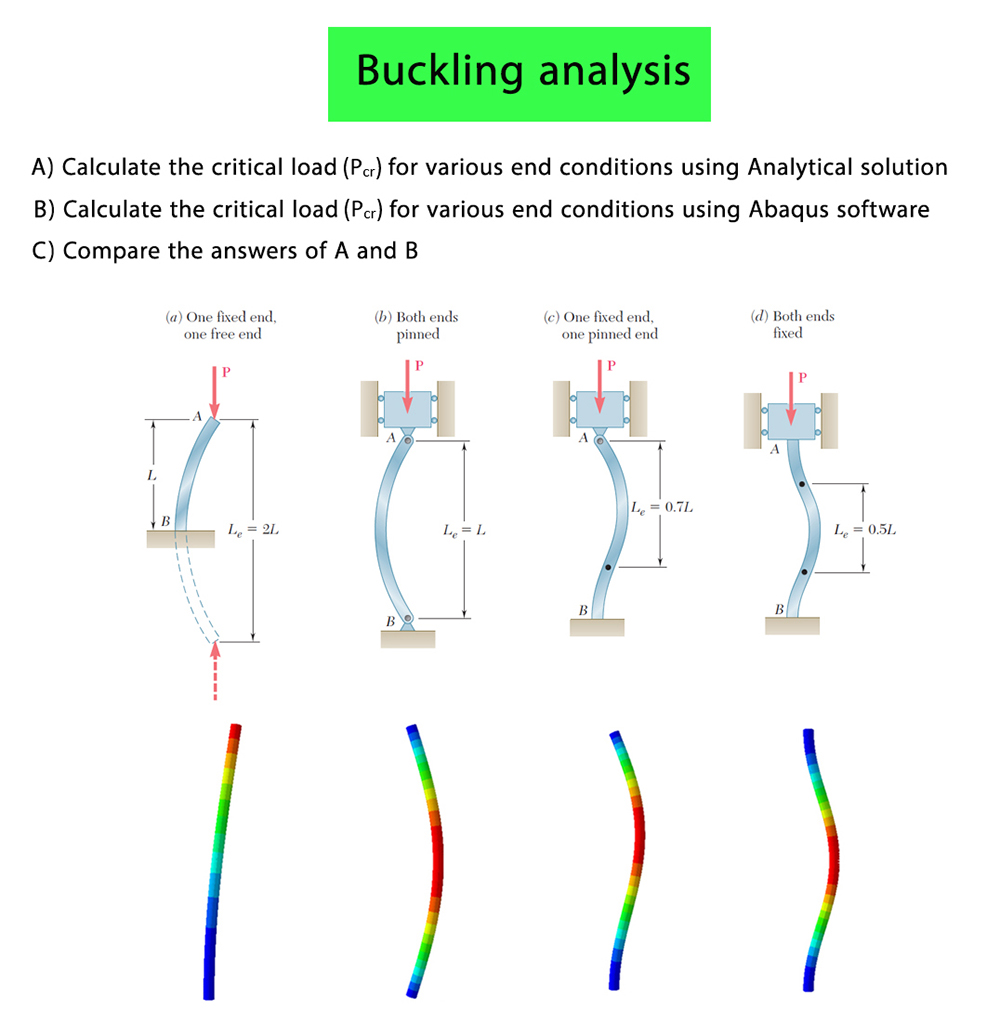The purpose of this simulation is to calculate the critical load using the Abaqus software and Analytical Solution and then compare the results obtained from the Abaqus software with those gained from the Analytical Solution

The height of column is equal to 1 m, The cross-section of the column is a circle with a radius equal to 0.02 m

Model (d) : Both ends fixed

In this case , the bottom of the column is absolutely fixed and the top section of the column can only move directed at Y axis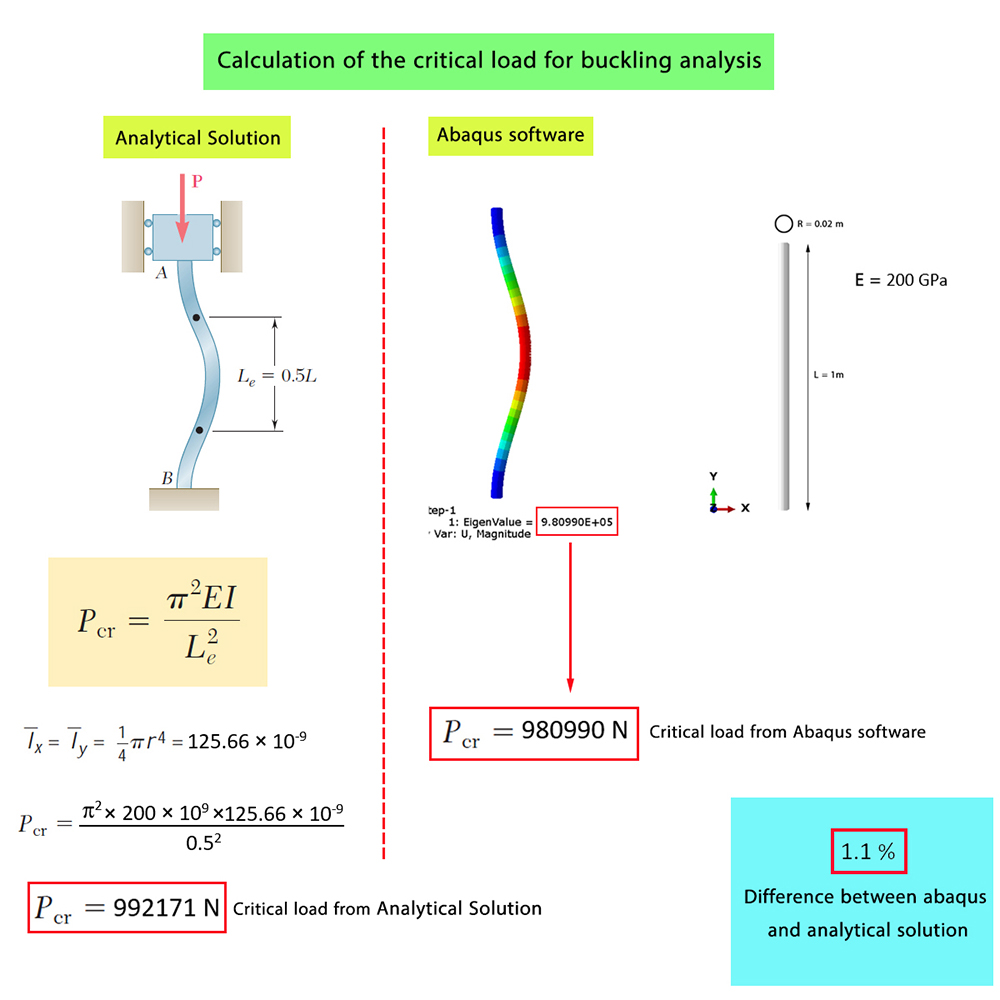Model (c) : One fixed end , one pinned end

In this case ,the bottom of the column absolutely fixed and at the top of the column the Hinged boundary condition has been used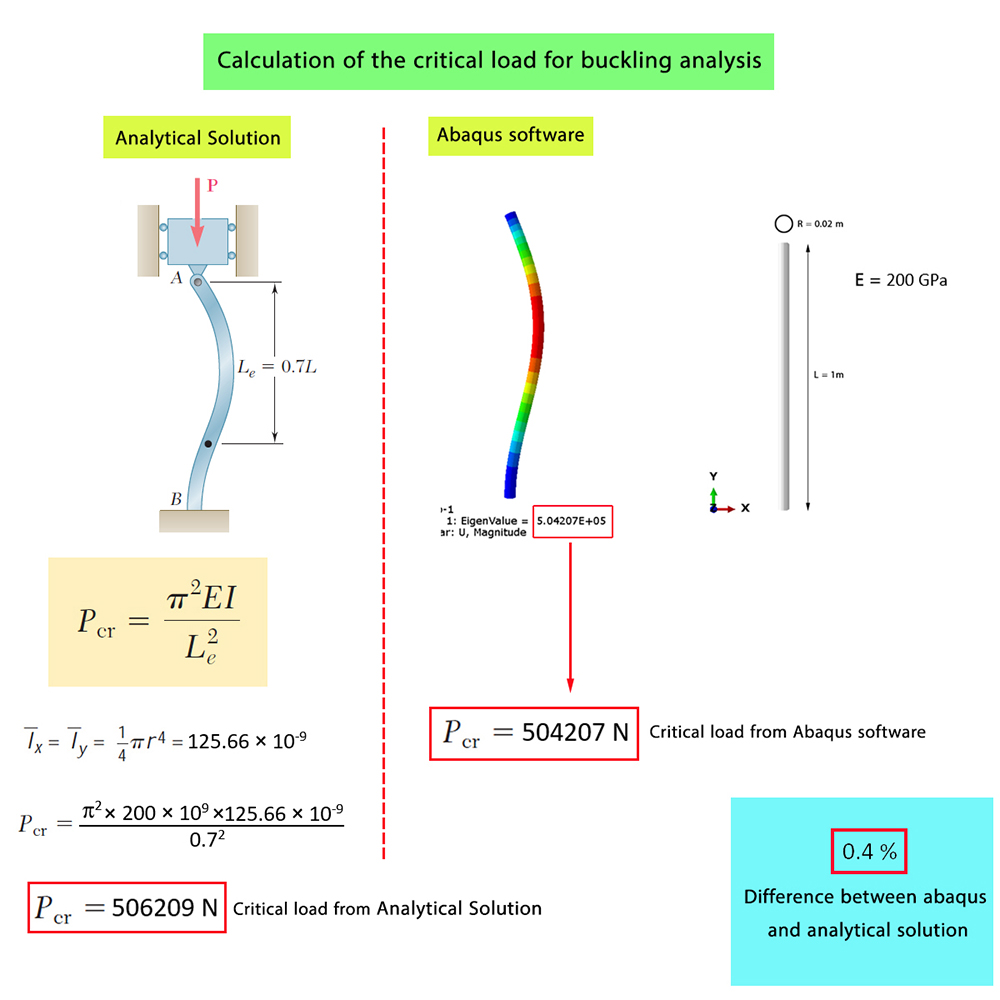Model (b) : Both ends pinned

In this case , top and bottom of the column is hinged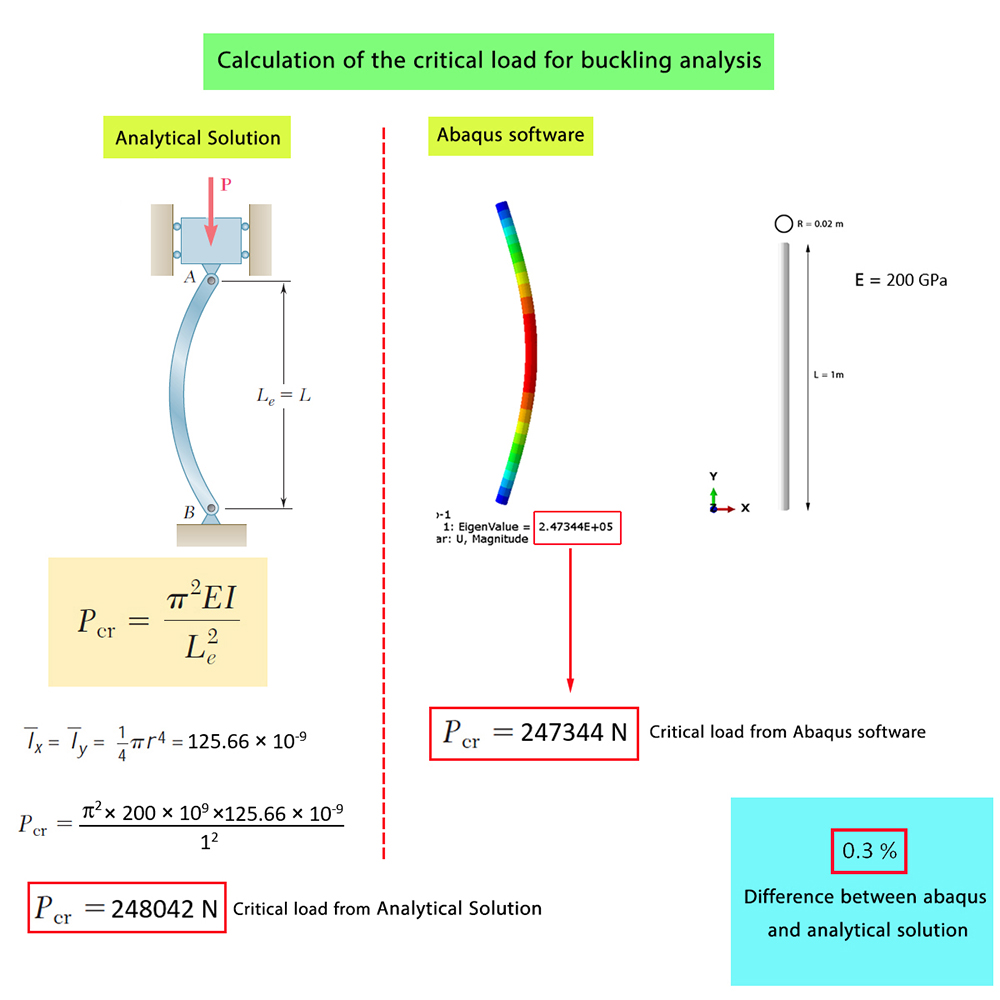Model (a) : One fixed end , one free end

In this case , the bottom of the column is quite fixed and the top section of the column is free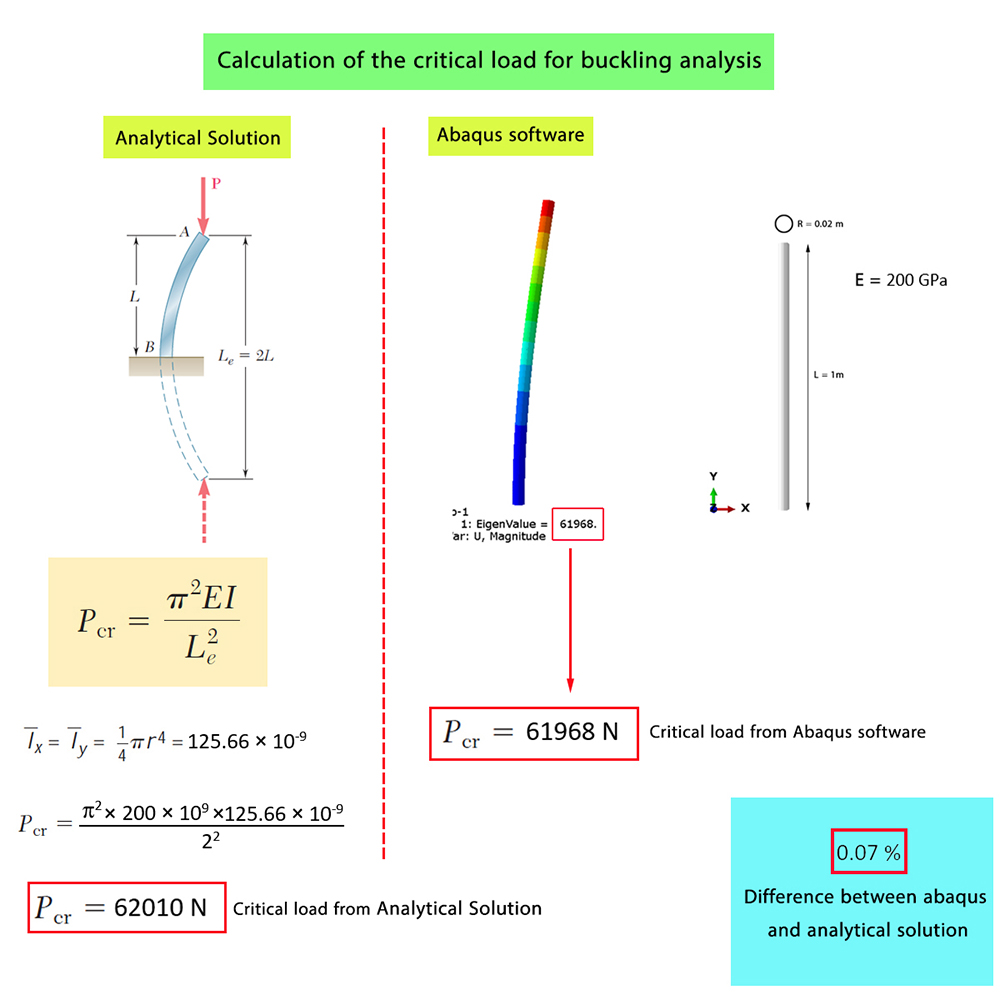As you observe, the results obtained from the Abaqus software are so close to those of Analytical Solution